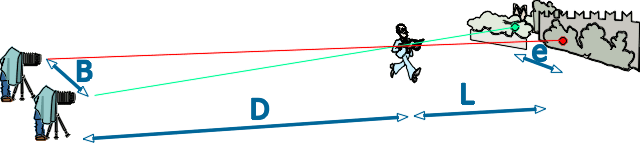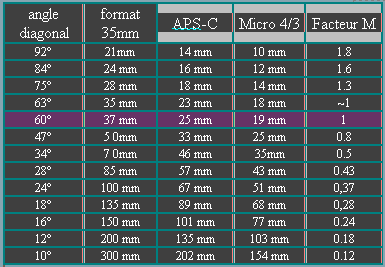3D  BASE  CALCULATIONS

 FOREWORD   The page on fundamentals explained why the stereoscopic effect is greater when the base is larger. The question is how to calculate the base depending on the camera and lens which is used. The human 3D vision is effective within 60° field. angle. Thus it is common sense to use as normal lenses giving the same field . Normally, the horizontal angle of field of the lens should be taken as the reference. But the angular field of lenses is often given as the diagonal field angle.  It is essential to choose carefully the desired stereoscopic ratio. See the tables SR / base and distances. Voir les tableaux SR / base sur la page correspondante.        1 < SR < 10  weak      10 < SR < 20  moderate      20 < SR < 35  heighten      35 < SR < 50  strong NB Hyper-stereoscopy is all relief which is over the normal visual stereoscopy. GENERAL FORMULA   The fundamental formula to calculate the base is B = SR . a . D (D+L) / L and is applicable to lenses and focal lengths giving  60° angle of field. SR   stereoscopic ratio (see Fundamentals page) a     eye keenness  a = 0.0004 D    distance from camera to subject L    distance from subject to backgroundCALCUL  et TABLES   Download the xls files MANUAL  CALCULATION    of  the    BASE Base calculation is rather simple with a programmable calculator, but we can do it with a simple calculator For lenses equivalent to 35-50 mm which angle of field range 55° to 45°, the factor 2.tan(A/2) below is taken equal to 1  Considering the formula, when expressing B in mm, D and L in meters, with k = 0.0004 we have a simplified formula B mm = SR . 0,4 . D . (1+t) / t   when t = L/D Let choose SR = 2.5 which is a low conventional stereoscopic effect: this is    B2,5  = D . (1+t) / t Now, the calculation is really simple: Calculate t = L/D then get (1+t) / t and multiply by D   Example: D = 30m and L = 90m, so t = 3 B2,5 = D .(1+3) / 3 = 30 . 4 / 3 = 40mm   For other stereoscopic ratio, increase the base proportionally. To obtain SR = 5  the base will be double B5 = 80mm CHANGING FOCAL LENGH To the general formula of the base, it should be added a factor when using different angle of field. In human vision the horizontal angle is between 50° and 60°. The reference for lenses will be 60° as diagonal and 53° as horizontal field angle thus the field factor is M = 2. tan(53°/2) =1  The factor of a lens with another field angle is M = 2 . tan (A/2) where A is the horizontal angle of field of the lens. B = 2 . tan(A/2) . SR . a . D (D+L) / L    Note: The diagonal field angle is the same whatever are the sensors APS-C, 4/3, and the format of the pictures, 3/2, 4/3,   etc. M varies around de 20% between 50° and 70°. Within this range apply M-=1 will not really change the stereoscopic ratio. Note  M = 2 . tan (A/2) = 37 / F* as with 35mm film Hereunder are M factors for common angles of field:MACROGRAPHY In macrography, the magnifying factor G is to be added: G = T / F with T extension and F focal length B = SR . a . D (D+L) / L / G VIEWING SYSTEMS The stereoscopic effect varies with the viewing system considering the image is viewed at the standard distance, or not, because it modifies the magnification of the picture. The factor to apply is cos 30° . Dp / Do where Dp is the diagonal of the picture Dy is the distance of the observer's eye to the picture. The formula is: SRy = SR . cos 30° . Dp / Dy cos 30° = 0.87